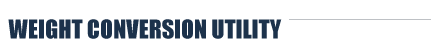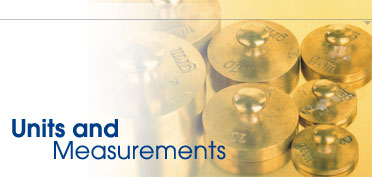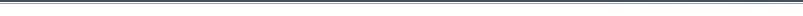Home > Weight Conversion UtilityWeight and Mass Conversion

 From: = To: atomic mass unit (amu) carat (metric) cental centigram dekagram dram (dr) grain (gr) gram (g) hundredweight (UK) kilogram (kg) microgram (µg) milligram (mg) newton (Earth) ounce (oz) pennyweight (dwt) pound (lb) quarter stone ton (UK, long) ton (US, short) tonne (t) troy ounce atomic mass unit (amu) carat (metric) cental centigram dekagram dram (dr) grain (gr) gram (g) hundredweight (UK) kilogram (kg) microgram (µg) milligram (mg) newton (Earth) ounce (oz) pennyweight (dwt) pound (lb) quarter stone ton (UK, long) ton (US, short) tonne (t) troy ounce
 This Weight and Mass Conversion program uses custom rounding routines to avoid results such as 3.9999999999 instead of 4.  It rounds the result to 13 significant digits instead of rounding to some number of decimal places, therefore producing superior results for very big or very small initial values and conversion factors.  It accepts scientific notation and converts immediately. Converting to and from: atomic mass unit (amu), carat (metric), cental, centigram, dekagram, dram (dr), grain (gr), gram (g), hundredweight (UK), kilogram (kg), microgram (µg), milligram (mg), newton (Earth), ounce (oz), pennyweight (dwt), pound (lb), quarter, stone, ton (UK, long), ton (US, short), tonne (t), troy ounce. Scripts used for weight and mass conversion are copyrights of Unit-conversion.info.